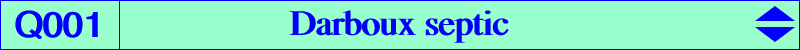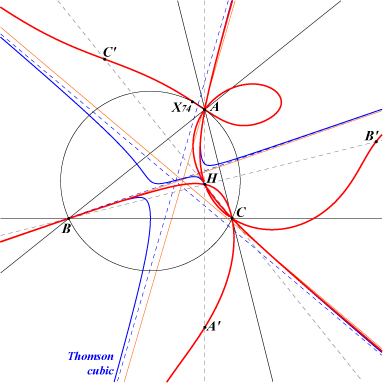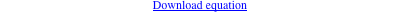too complicated to be written here. Click on the link to download a text file.A, B, C, H which are triple X(74), X(671), X(895), X(1156), X(1320), A', B', C' reflections of A, B, C in the sidelinesQ001 is a bicircular septic which solves the Darboux problem. See K172. It is the antigonal image of the Lucas cubic. (see Hyacinthos #8509-11-12-15 & sq.) and the isogonal transform of Q071. It has three real asymptotes parallel to those of the Lucas cubic or the Thomson cubic. The "last" point on BC is [0 : a^2 + 3(b^2 - c^2) : a^2 - 3(b^2 - c^2)], the other points on CA, AB similarly. These points are the vertices of the pedal triangle of X(3146), the homothetic of H under h(O,3). The tangents at A, B, C are the symmedians and the two other tangents at each vertex are not always real.